# Tensorflow入门1-CNN网络及MNIST例子讲解

+关注继续查看

## 1. 前言

• CNN的原理
• Tensorflow使用CNN网络解决MNIST问题的例子讲解
• Demo改造解决我自己的股票图片识别问题
• 训练出的模型部署到iOS端的Tensorflow Mobile框架中
• 训练出的模型部署到iOS端的Tensorflow List框架中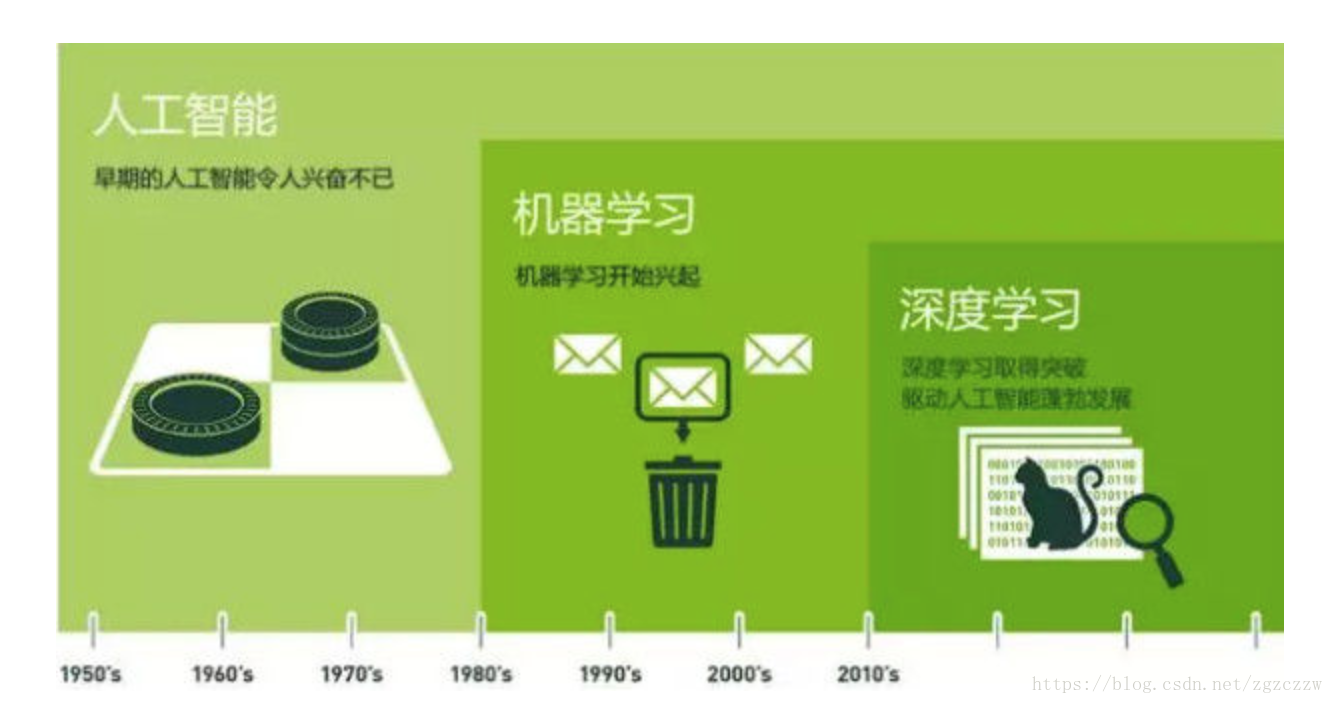## 2. CNN神经网络

CNN神经网络，全称是卷积神经网络，是目前深度学习中最常见、应用最广泛的一种网络，适合于解决图像识别，图片分类，图像预测的问题。

An Intuitive Explanation of Convolutional Neural Networks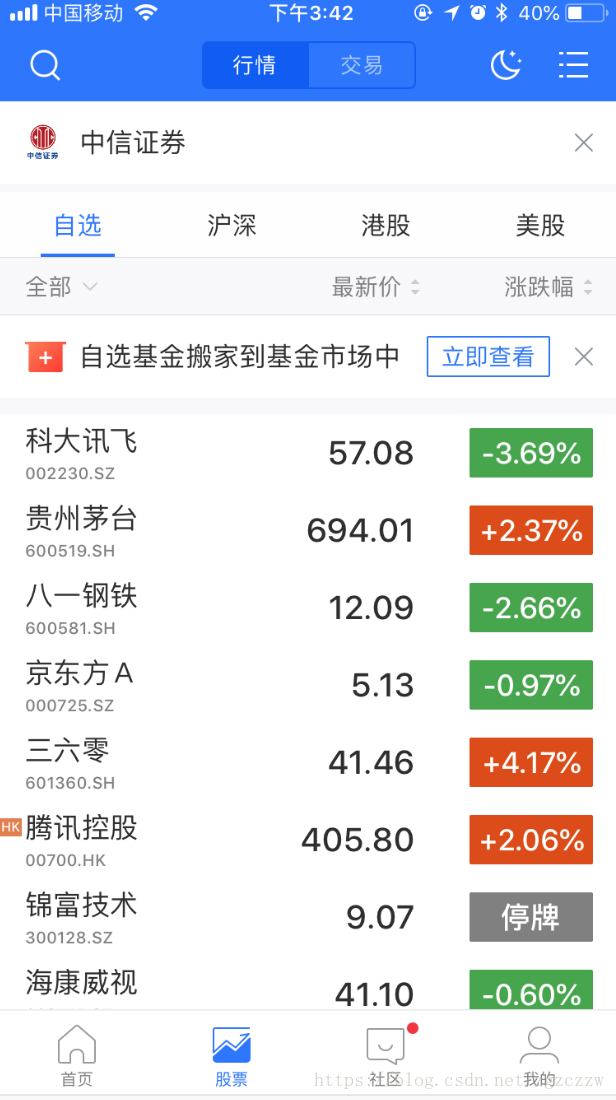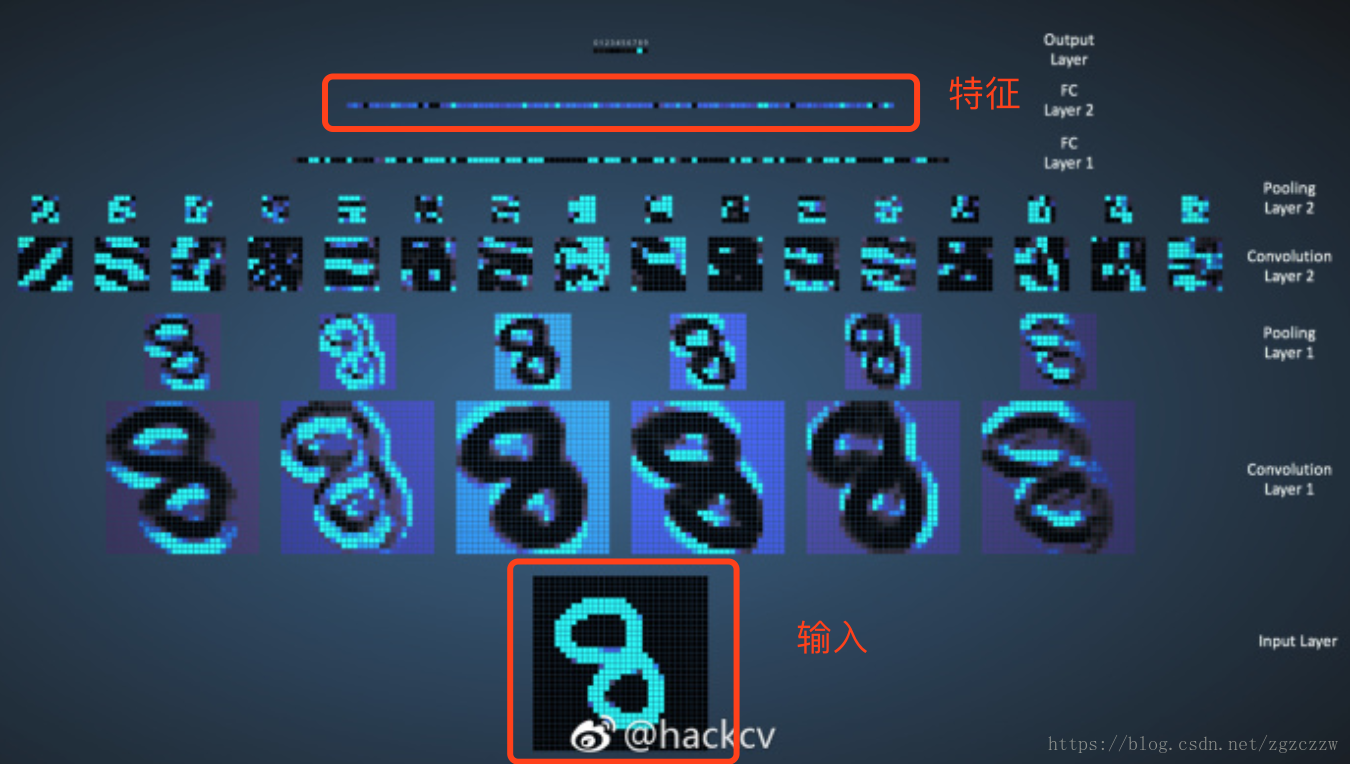特征1 -> 标签A

## 3. MNIST问题

MNIST问题就相当于图像处理的Hello World程序，在Tensorflow的官方教程里已经有一个完整的Demo。

MNIST问题处理

MNIST问题是很常见的图片分类问题，训练集是已经编码过的手写图片，图片中是手写的0~9的数字，模型经过训练后，输入一张图片，可以输出0~9的数字。

### 3.1 输入集

//训练集-图片数据
train-images-idx3-ubyte.gz:  training set images (9912422 bytes)
//训练集-label数据
train-labels-idx1-ubyte.gz:  training set labels (28881 bytes)
//测试集-图片数据
t10k-images-idx3-ubyte.gz:   test set images (1648877 bytes)
//测试集-label数据
t10k-labels-idx1-ubyte.gz:   test set labels (4542 bytes)

[offset] [type]          [value]          [description]
0000     32 bit integer  0x00000803(2051) magic number
0004     32 bit integer  60000            number of images
0008     32 bit integer  28               number of rows
0012     32 bit integer  28               number of columns
0016     unsigned byte   ??               pixel
0017     unsigned byte   ??               pixel
........
xxxx     unsigned byte   ??               pixel

[offset] [type]          [value]          [description]
0000     32 bit integer  0x00000801(2049) magic number (MSB first)
0004     32 bit integer  60000            number of items
0008     unsigned byte   ??               label
0009     unsigned byte   ??               label
........
xxxx     unsigned byte   ??               label
The labels values are 0 to 9.

#coding=utf-8
import os
import struct
import numpy as np
import matplotlib.pyplot as plt

# 读取文件
labels_path = os.path.join(path,
'%s-labels-idx1-ubyte'
% kind)
images_path = os.path.join(path,
'%s-images-idx3-ubyte'
% kind)
with open(labels_path, 'rb') as lbpath:
# 读取magic numer，labels数量
magic, n = struct.unpack('>II',
print 'label magic : ',
print magic
# 载入label数据
labels = np.fromfile(lbpath,
dtype=np.uint8).reshape(n, 1)

with open(images_path, 'rb') as imgpath:
# 读取magic numer，图片数量，图片宽，高
magic, num, rows, cols = struct.unpack('>IIII',
print 'image magic : ',
print magic
# 载入图片数据
images = np.fromfile(imgpath,
dtype=np.uint8).reshape(num, rows * cols)

return images, labels, rows, cols

def show_image():
images, labels, rows, cols = load_mnist('/tmp/tensorflow/mnist/input_data/')
fig, ax = plt.subplots(
nrows=2,
ncols=5,
sharex=True,
sharey=True, )

ax = ax.flatten()
for i in range(10):
img = images[i].reshape(rows, cols)
print labels[i]
ax[i].imshow(img, cmap='Greys', interpolation='nearest')

ax.set_xticks([])
ax.set_yticks([])
plt.tight_layout()
plt.show()

if __name__ == '__main__':
show_image()label magic :  2049
image magic :  2051
         

### 3.2 MNIST问题代码解析

#### 3.2.1 读取数据

if __name__ == '__main__':
# 解析命令行参数，默认没有
parser = argparse.ArgumentParser()
# 添加MNIST数据集的下载地址
default='/tmp/tensorflow/mnist/input_data',
help='Directory for storing input data')
FLAGS, unparsed = parser.parse_known_args()
# 运行main方法
tf.app.run(main=main, argv=[sys.argv] + unparsed)
  # Import data
mnist = input_data.read_data_sets(FLAGS.data_dir)

def read_data_sets(train_dir,
fake_data=False,
one_hot=False,
dtype=dtypes.float32,
reshape=True,
validation_size=5000,
seed=None,
source_url=DEFAULT_SOURCE_URL):
...
return base.Datasets(train=train, validation=validation, test=test)

#### 3.2.2 定义输入输出

  # Create the model
x = tf.placeholder(tf.float32, [None, 784], name="x")

# Define loss and optimizer
y_ = tf.placeholder(tf.int64, [None])


tf.placeholder可以理解为定义变量，这里定义了两个变量，一个是输入值x，类型是浮点数，纬度是[None, 784]，None代表不确定数量，784代表28*28，也就是一张MNIST输入集的图片，这里的意思是说输入层x可以是不确定数量的图片，代表着我们这个网络可以同时输入多张图片的数据。这种变量就是tensorflow的张量tensor，而每个tensor都可以指定名称，但是我们一般只指定具有代表性的几个tensor的名称，比如这个输入节点的名称是x。

#### 3.2.3 定义计算图

# Build the graph for the deep net
y_conv = deepnn(x)

deepnn这个方法是整个程序的核心，在这个方法中定义了一整个计算图。我们一层一层的看。这个CNN的网络用的是LeNet网络。构成是这样的。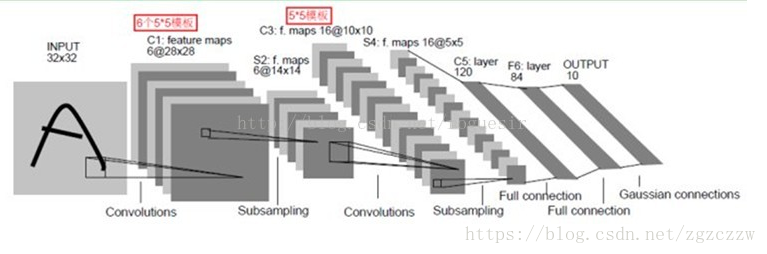def deepnn(x):
"""deepnn builds the graph for a deep net for classifying digits.

Args:
x: an input tensor with the dimensions (N_examples, 784), where 784 is the
number of pixels in a standard MNIST image.

Returns:
A tuple (y, keep_prob). y is a tensor of shape (N_examples, 10), with values
equal to the logits of classifying the digit into one of 10 classes (the
digits 0-9). keep_prob is a scalar placeholder for the probability of
dropout.
"""
# Reshape to use within a convolutional neural net.
# Last dimension is for "features" - there is only one here, since images are
# grayscale -- it would be 3 for an RGB image, 4 for RGBA, etc.
with tf.name_scope('reshape'):
x_image = tf.reshape(x, [-1, 28, 28, 1])

# First convolutional layer - maps one grayscale image to 32 feature maps.
with tf.name_scope('conv1'):
W_conv1 = weight_variable([5, 5, 1, 32])
b_conv1 = bias_variable()
h_conv1 = tf.nn.relu(conv2d(x_image, W_conv1) + b_conv1)

# Pooling layer - downsamples by 2X.
with tf.name_scope('pool1'):
h_pool1 = max_pool_2x2(h_conv1)

# Second convolutional layer -- maps 32 feature maps to 64.
with tf.name_scope('conv2'):
W_conv2 = weight_variable([5, 5, 32, 64])
b_conv2 = bias_variable()
h_conv2 = tf.nn.relu(conv2d(h_pool1, W_conv2) + b_conv2)

# Second pooling layer.
with tf.name_scope('pool2'):
h_pool2 = max_pool_2x2(h_conv2)

# Fully connected layer 1 -- after 2 round of downsampling, our 28x28 image
# is down to 7x7x64 feature maps -- maps this to 1024 features.
with tf.name_scope('fc1'):
W_fc1 = weight_variable([7 * 7 * 64, 1024])
b_fc1 = bias_variable()

h_pool2_flat = tf.reshape(h_pool2, [-1, 7 * 7 * 64])
h_fc1 = tf.nn.relu(tf.matmul(h_pool2_flat, W_fc1) + b_fc1)

# Map the 1024 features to 10 classes, one for each digit
with tf.name_scope('fc2'):
W_fc2 = weight_variable([1024, 10])
b_fc2 = bias_variable()

y_conv = tf.add(tf.matmul(h_fc1, W_fc2), b_fc2, name="output")
return y_conv

##### 卷积层

CNN叫卷积神经网络，可以看出卷积是这个网络的核心。卷积层是用于提取图片特征，卷积的操作是用一个卷积矩阵（也叫卷积核）在输入矩阵上依次扫描，做矩阵相乘，得到的结果输入矩阵的某一个特征。这样讲是不太好理解，下面用图片说一下这个过程。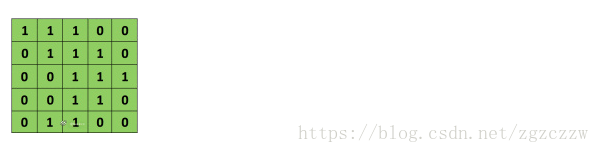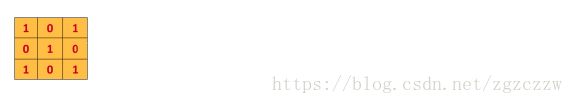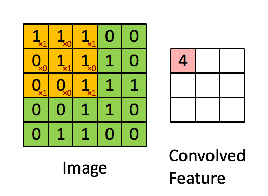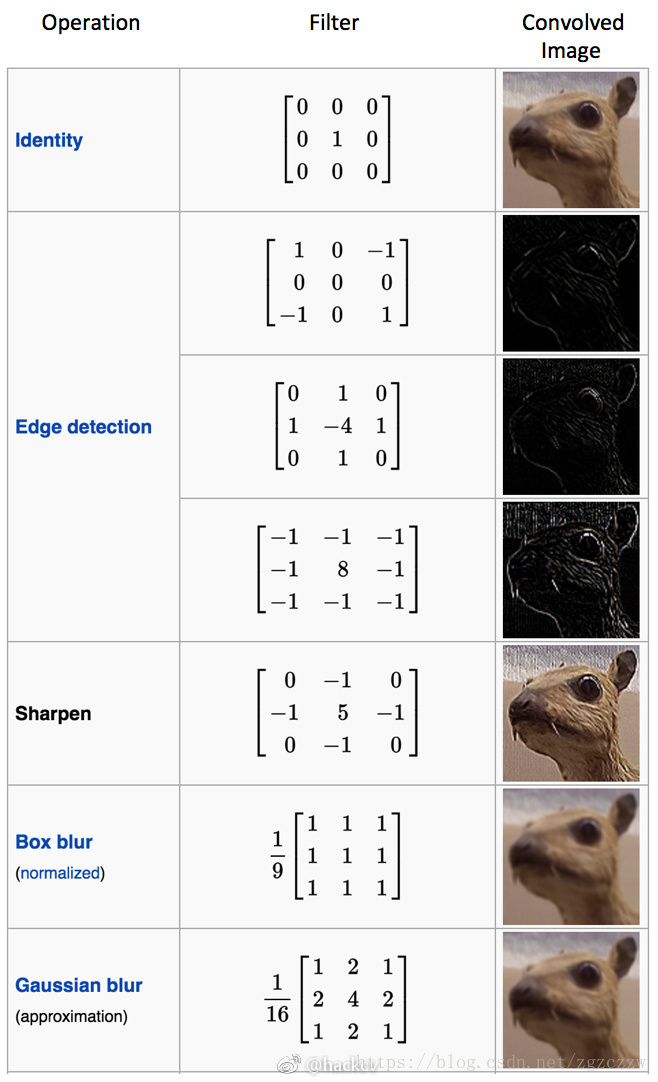# First convolutional layer - maps one grayscale image to 32 feature maps.
with tf.name_scope('conv1'):
W_conv1 = weight_variable([5, 5, 1, 32])
b_conv1 = bias_variable()
h_conv1 = tf.nn.relu(conv2d(x_image, W_conv1) + b_conv1)

def weight_variable(shape):
"""weight_variable generates a weight variable of a given shape."""
initial = tf.truncated_normal(shape, stddev=0.1)
return tf.Variable(initial)

tf.Variable生成一个变量tensor，参数initial是初始化值。initial由truncated_normal方法生成，shape指定了生成变量的纬度，这里是[5,5,1,32]四维的变量，初始化值是由truncated_normal产生的正态分布的值，这就是truncated_normal方法的作用，详细的说明可以看文档。

def bias_variable(shape):
"""bias_variable generates a bias variable of a given shape."""
initial = tf.constant(0.1, shape=shape)
return tf.Variable(initial)

conv2d(x_image, W_conv1)

def conv2d(x, W):
"""conv2d returns a 2d convolution layer with full stride."""
return tf.nn.conv2d(x, W, strides=[1, 1, 1, 1], padding='SAME')

tf.nn.conv2d(input, filter, strides, padding, use_cudnn_on_gpu=None, name=None)

###### 二维特征向量

conv2d生成的是二维特征向量，conv2d有两个参数是必要的，input和filter。我们的输入参数是一张图片，三维的数据[宽，高，颜色空间]，为了最后向量相乘的结果是二维的，filer的第三个纬度应该和input的第四个纬度相等，也就是in_channels相等，这样永远都只能输出一个二维的特征向量，也就是这个函数叫conv2d的原因，这里比较难理解，可以仔细考虑一下。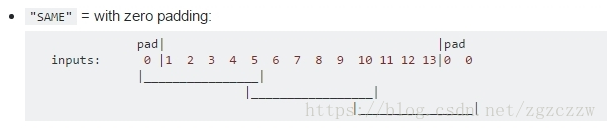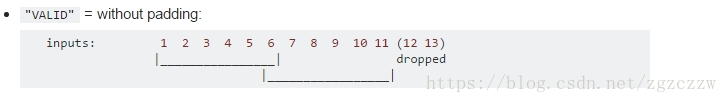W_conv1 = weight_variable([5, 5, 3, 32])
conv2d(x_image, W_conv1)

# 第一个卷积层，从图像中提取32的特征
with tf.name_scope('conv1'):
W_conv1 = weight_variable([5, 5, 3, 32])  # y =wX+b
b_conv1 = bias_variable()
h_conv1 = tf.nn.relu(conv2d(x_image, W_conv1) + b_conv1)

ReLU 是一个元素级别的操作（应用到各个像素），并将特征图中的所有小于 0 的像素值设置为零。ReLU 的目的是在 ConvNet 中引入非线性，因为在大部分的我们希望 ConvNet 学习的实际数据是非线性的（卷积是一个线性操作——元素级别的矩阵相乘和相加，所以我们需要通过使用非线性函数 ReLU 来引入非线性。

##### 池化

# Pooling layer - downsamples by 2X.
with tf.name_scope('pool1'):
h_pool1 = max_pool_2x2(h_conv1)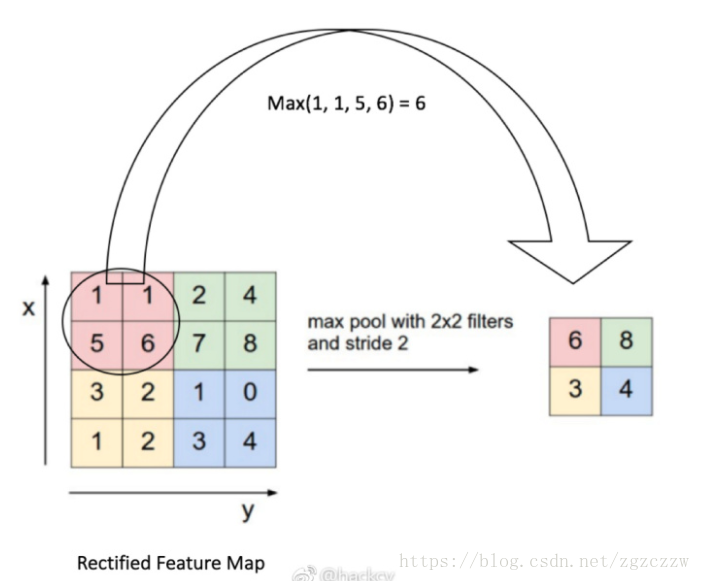# 2*2的最大化池化层
with tf.name_scope('pool1'):
h_pool1 = max_pool_2x2(h_conv1)
def max_pool_2x2(x):
"""max_pool_2x2 downsamples a feature map by 2X."""
return tf.nn.max_pool(x, ksize=[1, 2, 2, 1],
strides=[1, 2, 2, 1], padding='SAME')

# Second convolutional layer -- maps 32 feature maps to 64.
with tf.name_scope('conv2'):
W_conv2 = weight_variable([5, 5, 32, 64])
b_conv2 = bias_variable()
h_conv2 = tf.nn.relu(conv2d(h_pool1, W_conv2) + b_conv2)

# Second pooling layer.
with tf.name_scope('pool2'):
h_pool2 = max_pool_2x2(h_conv2)

##### 全连接

  # Fully connected layer 1 -- after 2 round of downsampling, our 28x28 image
# is down to 7x7x64 feature maps -- maps this to 1024 features.
with tf.name_scope('fc1'):
W_fc1 = weight_variable([7 * 7 * 64, 1024])
b_fc1 = bias_variable()

h_pool2_flat = tf.reshape(h_pool2, [-1, 7 * 7 * 64])
h_fc1 = tf.nn.relu(tf.matmul(h_pool2_flat, W_fc1) + b_fc1)

# Map the 1024 features to 10 classes, one for each digit
with tf.name_scope('fc2'):
W_fc2 = weight_variable([1024, 10])
b_fc2 = bias_variable()

y_conv = tf.add(tf.matmul(h_fc1, W_fc2), b_fc2, name="output")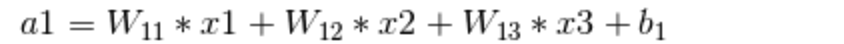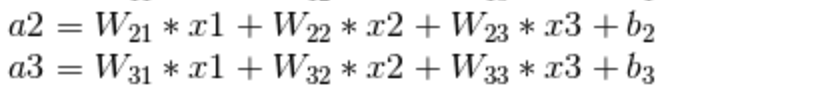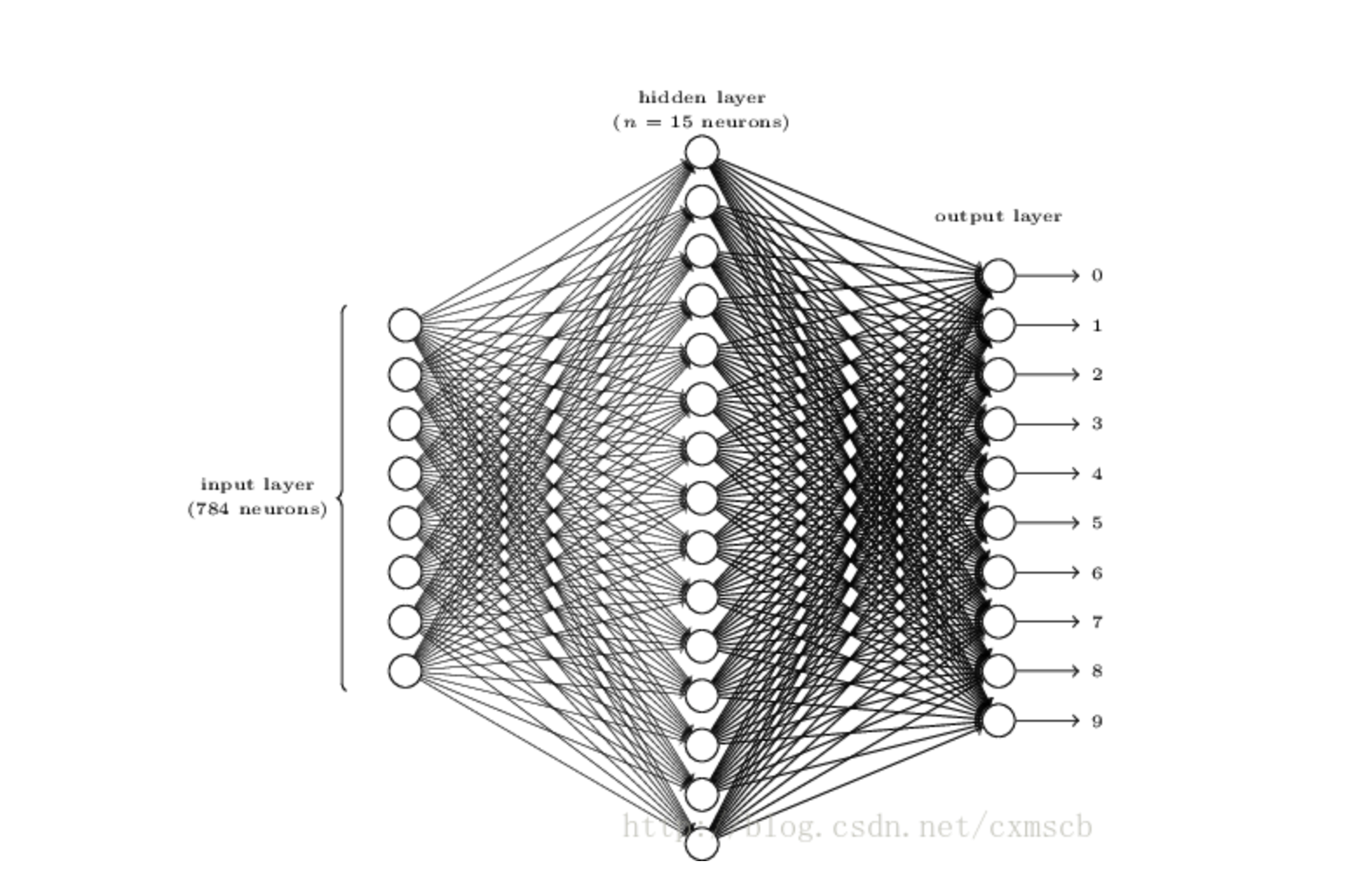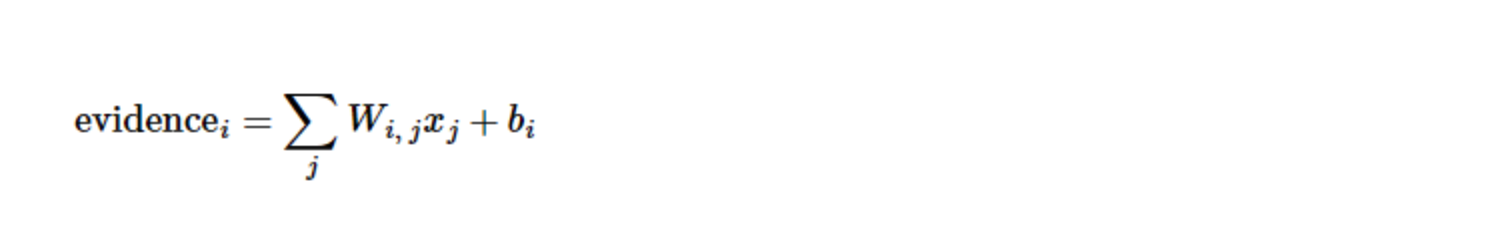# Fully connected layer 1 -- after 2 round of downsampling, our 28x28 image
# is down to 7x7x64 feature maps -- maps this to 1024 features.
with tf.name_scope('fc1'):
W_fc1 = weight_variable([7 * 7 * 64, 1024])
b_fc1 = bias_variable()

h_pool2_flat = tf.reshape(h_pool2, [-1, 7 * 7 * 64])
h_fc1 = tf.nn.relu(tf.matmul(h_pool2_flat, W_fc1) + b_fc1)

##### droupout

  # Dropout - controls the complexity of the model, prevents co-adaptation of
# features.
with tf.name_scope('dropout'):
keep_prob = tf.placeholder(tf.float32)
h_fc1_drop = tf.nn.dropout(h_fc1, keep_prob)

droupout的操作如下图所示：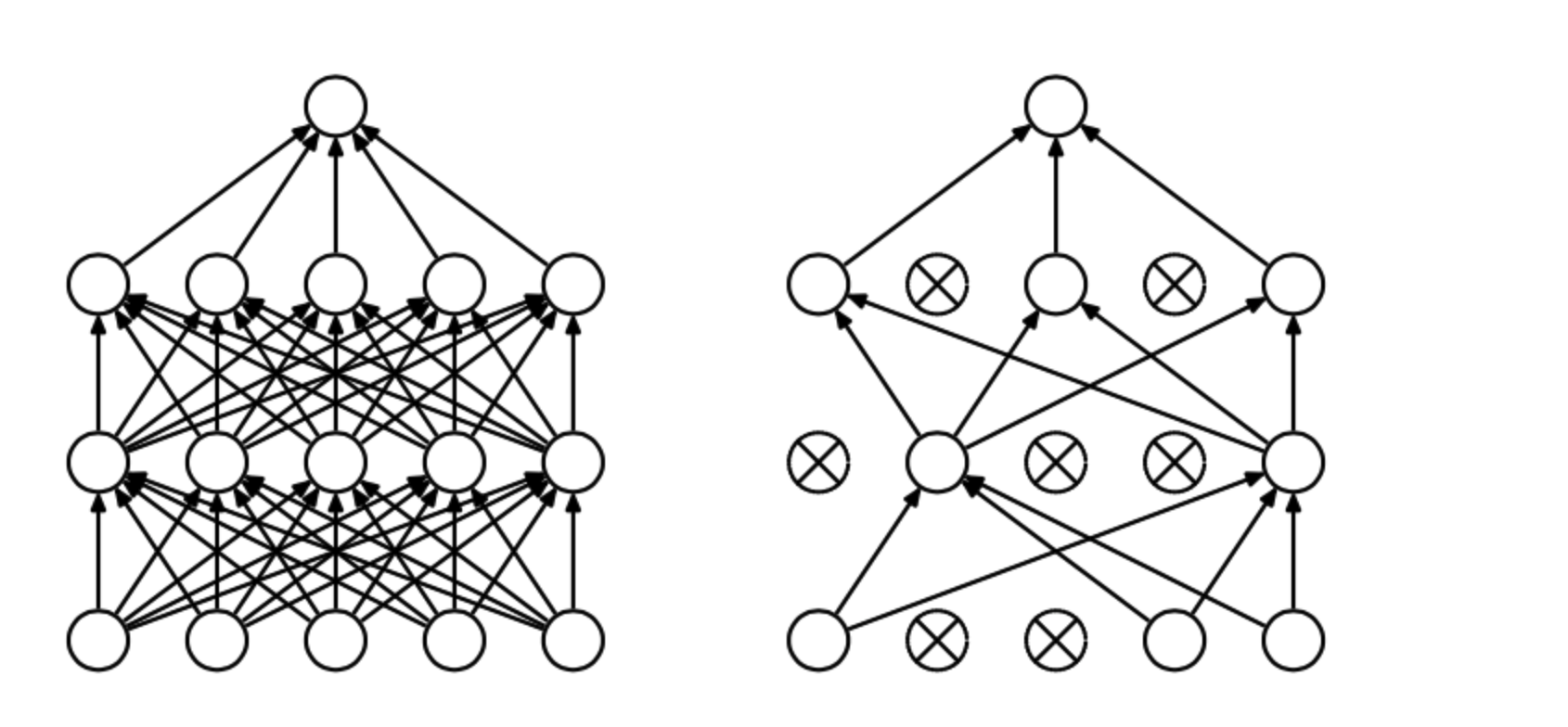• 解决过拟合的问题
• 在训练中加入概率性

droupout操作之后又是一层全连接。

  # Map the 1024 features to 10 classes, one for each digit
with tf.name_scope('fc2'):
W_fc2 = weight_variable([1024, 10])
b_fc2 = bias_variable()

y_conv = tf.add(tf.matmul(h_fc1, W_fc2), b_fc2, name="output")

#### 3.2.4 Softmax回归处理

  with tf.name_scope('loss'):
cross_entropy = tf.losses.sparse_softmax_cross_entropy(
labels=y_, logits=y_conv)
cross_entropy = tf.reduce_mean(cross_entropy)#### 3.2.5 Softmax回归处理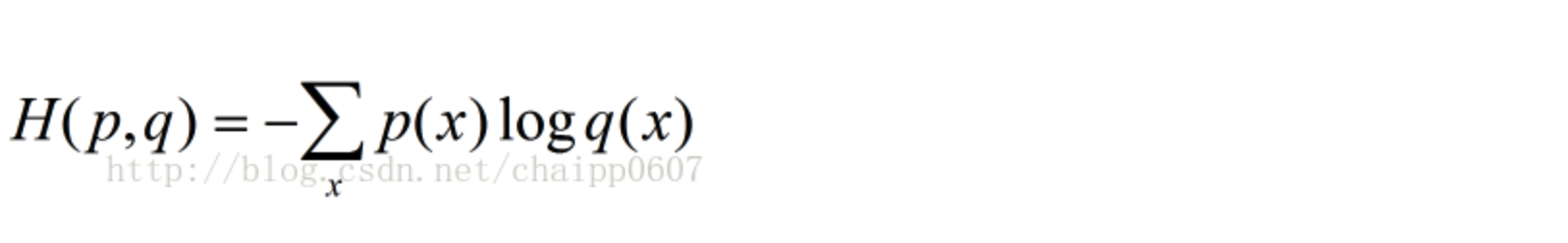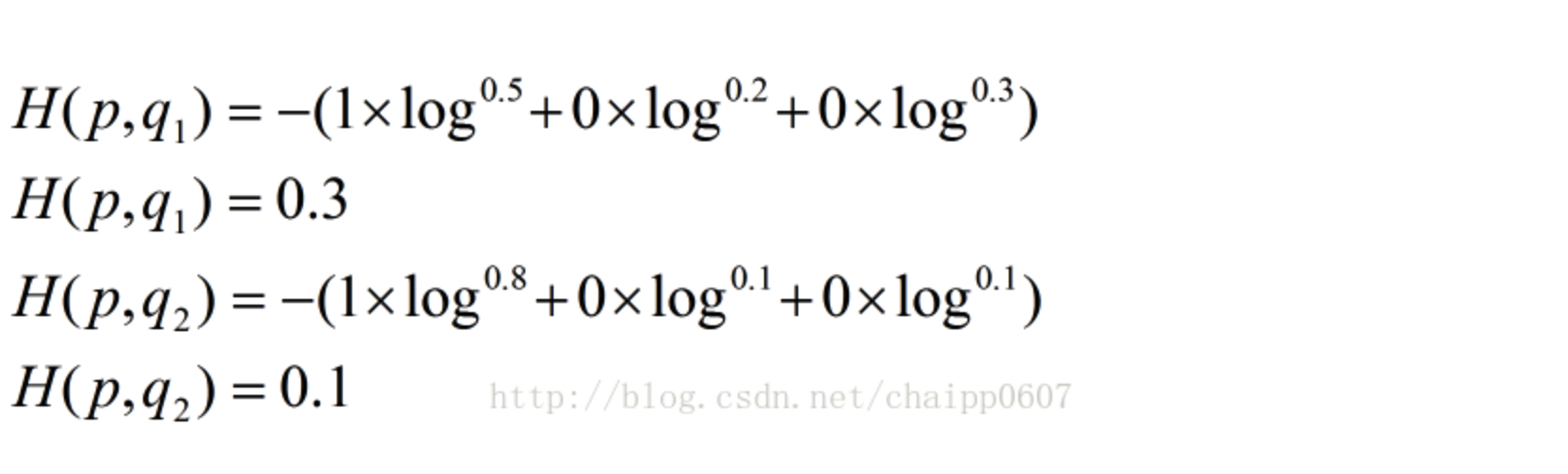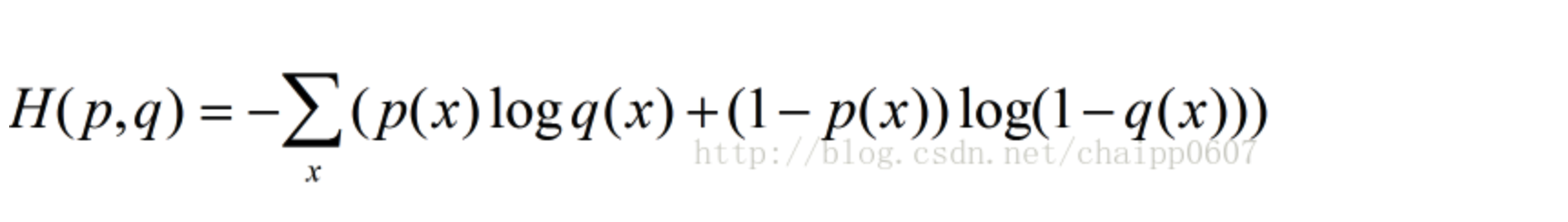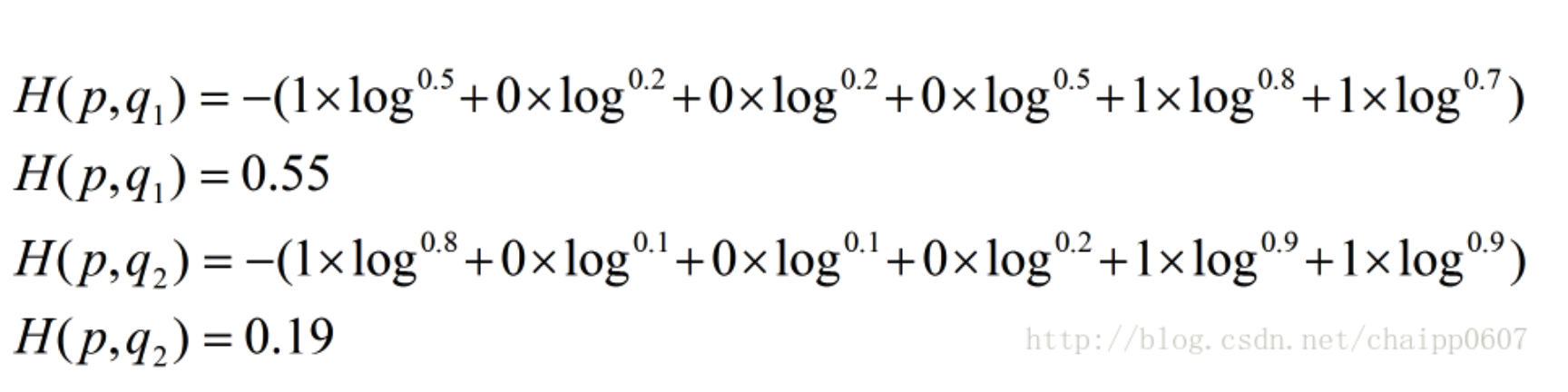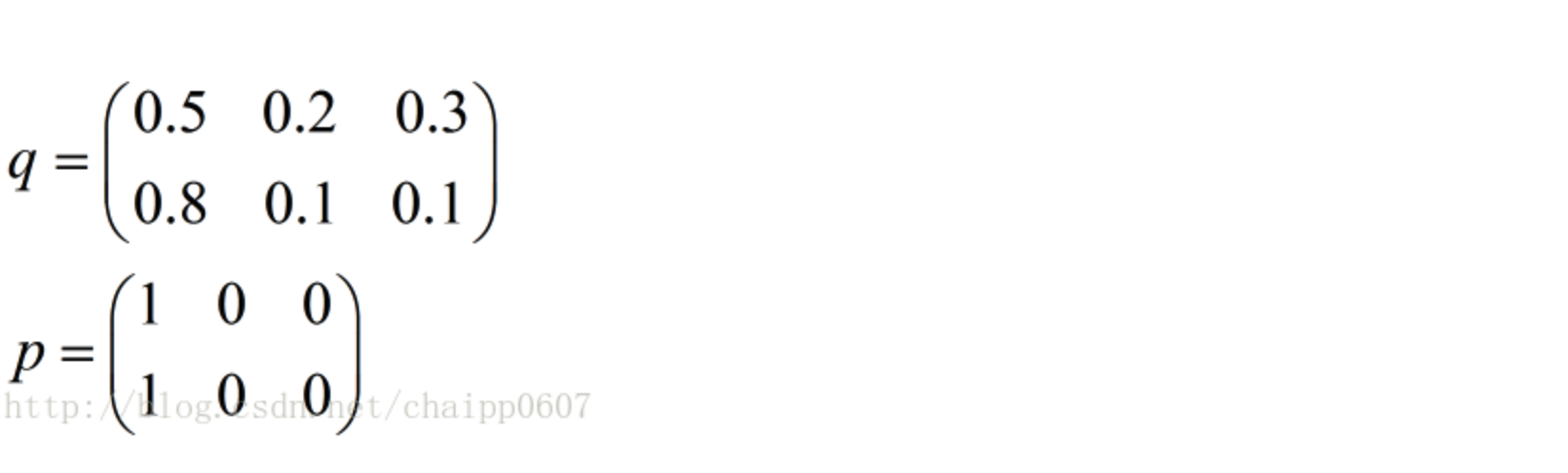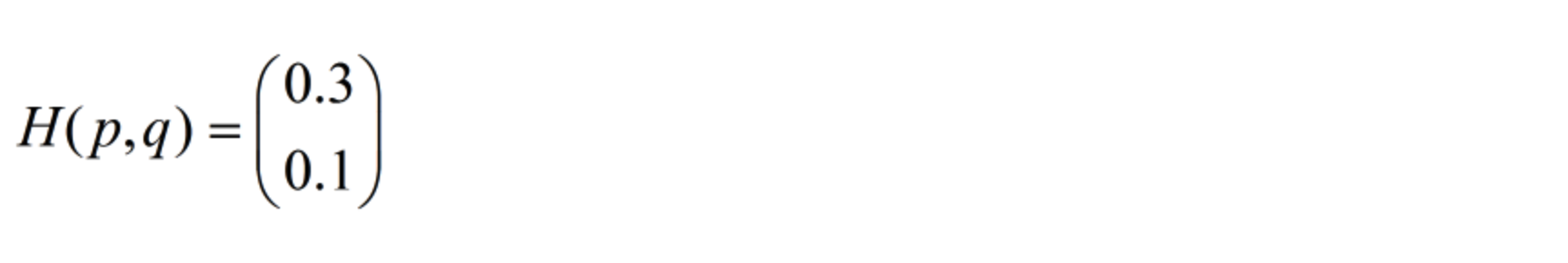tf.losses.sparse_softmax_cross_entropy是Tensorflow将以上两个过程封装后的产物，最后的结果是一个batch的概率值，reduce_mean用来取得概率的平均值。综上作为整个网络的损失函数。

#### 3.2.6 训练方法-梯度下降

  with tf.name_scope('adam_optimizer'):
train_step = tf.train.AdamOptimizer(1e-4).minimize(cross_entropy)

#### 3.2.7 准确度

  with tf.name_scope('accuracy'):
correct_prediction = tf.equal(tf.argmax(y_conv, 1), y_)
correct_prediction = tf.cast(correct_prediction, tf.float32)
accuracy = tf.reduce_mean(correct_prediction)

y_conv是网络输出的原始结果，也就是图片在最后10个节点上的权重值，比如[314, -423, 342...]，最后权重值最大的节点是我们的期望节点，比如这里第三个节点的权重值最大，那这幅图片可能就是数字2，tf.argmax是取出权重值最大的下标。y_是我们给的label值，把这两个值相比较得出准确度。最后依然用tf.reduce_mean算出batch的平均准确度。

#### 3.2.8 计算图可视化

  graph_location = tempfile.mkdtemp()
print('Saving graph to: %s' % graph_location)
train_writer = tf.summary.FileWriter(graph_location)
train_writer.add_graph(tf.get_default_graph())

#### 3.2.9 启动计算图

  with tf.Session() as sess:
sess.run(tf.global_variables_initializer())
for i in range(20000):
batch = mnist.train.next_batch(50)
if i % 100 == 0:
train_accuracy = accuracy.eval(feed_dict={
x: batch, y_: batch, keep_prob: 1.0})
print('step %d, training accuracy %g' % (i, train_accuracy))
train_step.run(feed_dict={x: batch, y_: batch, keep_prob: 0.5})

print('test accuracy %g' % accuracy.eval(feed_dict={
x: mnist.test.images, y_: mnist.test.labels, keep_prob: 1.0}))

sess.run(tf.global_variables_initializer())

train_step.run(feed_dict={x: batch, y_: batch, keep_prob: 0.5})

if i % 100 == 0:
train_accuracy = accuracy.eval(feed_dict={
x: batch, y_: batch, keep_prob: 1.0})
print('step %d, training accuracy %g' % (i, train_accuracy))

print('test accuracy %g' % accuracy.eval(feed_dict={
x: mnist.test.images, y_: mnist.test.labels, keep_prob: 1.0}))

20000次训练之后，输出最终的准确率。

step 0, training accuracy 0.16
step 100, training accuracy 0.9
step 200, training accuracy 0.94
step 300, training accuracy 0.9
step 400, training accuracy 0.96
...
test accuracy 0.9446

## 总结unix网络编程之简介
692 01588 01657 0《UNIX网络编程 卷2：进程间通信（第2版）》——1.8　书中IPC例子索引表

1059 0CNNdroid：在 Android 上利用 GPU 加速执行 CNN (卷积神经网络)
1393 0976 08270 0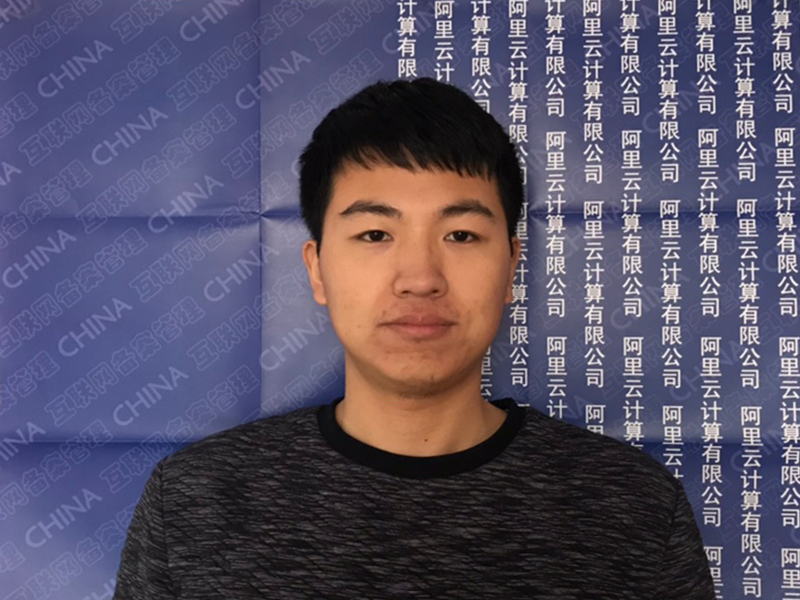Tensorflow入门1-CNN网络及MNIST例子讲解
## 1. 前言 人工智能自从阿尔法狗大败李世石后就异常火爆，最近工作中需要探索AI在移动端的应用，趁着这个计划入门下深度学习吧。 深度学习的入门曲线还是很陡峭的，看了很多资料，询问了从事相关工作的朋友后终于有点感觉了，这篇文章就讲一下我在这个过程中的所见所得吧，肯定是不专业的，如果所说有什么错误，也希望大家帮忙指出，共同进步。 这个入门的文章准备分以下几个部分来说： - CN
6305 02017年蜂窝网络发展趋势预测
1175 0868 0

ios开发工程师
5

0

《SaaS模式云原生数据仓库应用场景实践》

《看见新力量：二》电子书